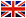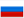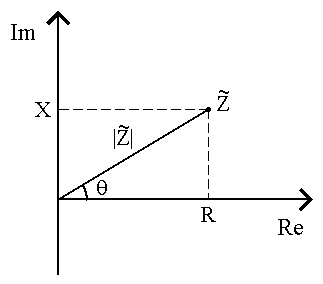Time of Flight Secondary Ion Mass Spectrometr xyz-table control## Avaliable methods of research

### Impedance Spectroscopy

Electrical impedance, or simply impedance, describes a measure of opposition to a sinusoidal alternating current (AC).
Electrical impedance extends the concept of resistance to AC circuits, describing not only the relative amplitudes of the voltage and current, but also the relative phases. In general impedance is a complex quantity Z and the term complex impedance may be used interchangeably; the polar form conveniently captures both magnitude and phase characteristics,

Z  =  Z  ej*phi

where the magnitude Z gives the change in voltage amplitude for a given current amplitude, while the argument phi gives the phase difference between voltage and current. In Cartesian form,

Z  =  R  +  j*X

where the real part of impedance is the resistance R and the imaginary part is the reactance X.

Resistance is the real part of impedance; a device with a purely resistive impedance exhibits no phase shift between the voltage and current.

Reactance is the imaginary part of the impedance; a component with a finite reactance induces a phase shift phi between the voltage across it and the current through it.Note that while reactance X can be either positive or negative, resistance R is always positive.

• Frequency 10-3 – 106 Hz

• Amplitude 10mV - 300mV

• Bias 0mV - 2V

... top